# Bathtub

Kevin has a bathtub shaped like a block. The bathtub measures 70 cm long, 45 cm wide and 50 cm high. Then the bathtub will be filled with clean water 3/5 of its height. The clean water needed is….

V =  94.5 l

### Step-by-step explanation: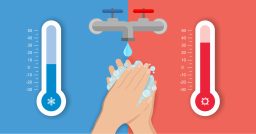Did you find an error or inaccuracy? Feel free to write us. Thank you!Tips to related online calculators
Need help to calculate sum, simplify or multiply fractions? Try our fraction calculator.
Do you know the volume and unit volume, and want to convert volume units?

## Related math problems and questions:

• Water tank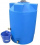The block-shaped tank is 2.5 m long, 100 cm wide and 12 dm high. In how many minutes will it be filled with water to two thirds if 40 liters of water flow into the tank per minute?
• Aquarium 6How high is the water level in the aquarium with a rectangular base 40cm and 50cm if it is filled 0,65hl of water?
• Cylinder-shaped vase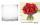If the cylinder-shaped vase is filled with water up to 35 cm, it contains 1 liter of water. How much water will it contain if it is filled to a height of 45cm?
• AquariumThe box-shaped aquarium is 40 cm high; the bottom has dimensions of 70 cm and 50 cm. Simon wanted to create an exciting environment for the fish, so he fixed three pillars to the bottom. They all have the shape of a cuboid with a square base. The base edg
• Water blockA block with a 50 cm2 base is filled with water 5 cm under the edge. How many can sugar cubes with 2 cm edge be thrown into a container that overflow water?
• Glass aquarium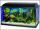How many m2 of glass are needed to produce an aquarium with bottom dimensions of 70 cm x 40 cm and 50 cm high?
• Water level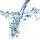To cuboid-shaped poll, bottom size 2m and 3.5m; flows water at a rate of 50 liters per minute. How long will it take for water to reach level 50 cm?
• Aquarium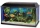I have an aquarium that is 100 cm long and 40 cm wide and 40 cm in height. We fill it with water. How much will it weigh?
• Cube-shaped containerThe cube-shaped container has a height of 52 cm and a square base. The container was filled to the brim with water, then we immersed a metal cube in it, which caused 2.7 l of water to flow out of the container. After removing the cube from the water, the
• Water tankWhat is the height of the cuboid-shaped tank with the bottom dimensions of 80 cm and 50 cm if the 480 liters of water reach 10 cm below the top?
• Swimming pool 4The pool shaped cuboid measuring 12.5 m × 640 cm at the bottom is 960hl water. To what height in meters reaches the water level?
• The aquariumThe aquarium is 120 cm long, 70 cm wide and 96 cm high. How high does the water reach if they filled 168l of water into the aquarium?
• Tank full2 hl of water were poured into a block-shaped tank with dimensions of 450 mm and 600 mm and a height of 900 mm. How many liters of water need to be added to keep the tank full?
• AquariumTry to estimate the weight of the water in an aquarium 50cm long 30cm wide, when poured to a height of 25cm. Calculates the weight of the aquarium's water.
• PoolThe swimming pool is 10 m wide and 8 m long, and 153 cm deep. How many hectoliters of water are in it if the water is 30 cm below its upper edge?
• AquariumAquarium is rectangular box with square base containing 76 liters of water. Length of base edge is 42 cm. To what height the water level goes?
• Children's poolChildren's pool at the swimming pool is 10m long, 5m wide and 50cm deep. Calculate: (a) how many m2 of tiles are needed for lining the perimeter walls of the pool? (b) how many hectoliters of water will fit into the pool?